• 关系运算符
千次阅读
2021-03-02 11:06:19

关系运算符

开发工具与关键技术：eclipse java
作者： 梁峻豪
撰写时间：2021-02-28


关系运算符（relational operators）也可以称为“比较运算符”，用于用来比较判断两个变量
或常量的大小。关系运算符是二元运算符，运算结果是 boolean 型。当运算符对应的关系
成立时，运算结果是 true，否则是 false。
关系表达式是由关系运算符连接起来的表达式。关系运算符中“关系”二字的含义是指一个
数据与另一个数据之间的关系，这种关系只有成立与不成立两种可能情况，可以用逻辑值
来表示，逻辑上的 true 与 false 用数字 1 与 0 来表示。关系成立时表达式的结果为 true（
或 1），否则表达式的结果为 false（或 0）。表 1 给出了比较运算符的含义及其实例应用

运算符 含义 说明 实例 结果

大于运算

只支持左右两边操作数是数值类型。如果前面变
量的值大于后面变量的值， 则返回 true。
2>3 false

=
大于或等
于运算符
只支持左右两边操作数是数值类型。如果前面变
量的值大于等于后面变量的值， 则返回 true。
4>=2 true
<
小于运算

只支持左右两边操作数是数值类型。如果前面变
量的值小于后面变量的值，则返回 true。
2<3 true
<=
小于或等
于运算符
只支持左右两边操作数是数值类型。如果前面变
量的值小于等于后面变量的值， 则返回 true。
4<=2 false
==
相等运算

如果进行比较的两个操作数都是数值类型，无论
它们的数据类型是否相同，只要它们的值不相等
，也都将返回 true。
如果两个操作数都是引用类型，只有当两个引用
变量的类型具有父子关系时才可以比较，只要两
个引用指向的不是同一个对象就会返回 true。
java 也支持两个 boolean 类型的值进行比较。
44
97
’a’
5.05
true
fals
e
true
true
true
false
!=
不相等运
算符
如果进行比较的两个操作数都是数值类型，无论
它们的数据类型是否相同，只要它们的值不相等
，也都将返回 true。
如果两个操作数都是引用类型，只有当两个引用
变量的类型具有父子关系时才可以比较，只要两
4!=2 true
个引用指向的不是同一个对象就会返回 true。
注意点如下所示：
基本类型的变量、值不能和引用类型的变量、值使用 == 进行比较；boolean 类型的
变量、值不能与其他任意类型的变量、值使用 == 进行比较；如果两个引用类型之间没有
父子继承关系，那么它们的变量也不能使用 == 进行比较。
== 和 != 可以应用于基本数据类型和引用类型。当用于引用类型比较时，比较的是
两个引用是否指向同一个对象，但当时实际开发过程多数情况下，只是比较对象的内容是
否相当，不需要比较是否为同一个对象。
关系运算符的优先级为：>、<、>=、<= 具有相同的优先级，并且高于具有相同优先级的 !=
。关系运算符的优先级高于赋值运算符而低于算术运算符，结合方向是自左向右。
关系表达式通常用于 Java 程序的逻辑判断语句的条件表达式中。使用关系表达式要注意以
下几点：
运算符 >=、
、！=、<= 是两个字符构成的一个运算符，用空格从中分开写就会产
生语法错误。例如 x> =y; 是错误的，但是可以写成 x >= y; 在运算符的两侧增加空格会提高
可读性。同样将运算符写反，例如 =>、=<、=! 等形式会产生语法错误。
由于计算机内存放的实数与实际的实数存在着一定的误差，如果对浮点数进行 ==
（相等）或 !=（不相等）的比较，容易产生错误结果，应该尽量避免。
不要将“==”写成“=”。
下面是一些使用关系运算符的示例。
a > b // 比较变量 a 的值是否大于变量 b 的值
x+y> = z // 比较变量 x 与变量 y 的和是否大于或等于变量 z 的值
width * width+size != area // 比较变量 width 的平方加上变量 size 的值是否与变量
area 的值不相等
name == “zhht” // 比较变量 name 的值是否等于字符串 nzht
pass != “123456” // 比较变量 pass 的值是否不等于字符串“123456”

更多相关内容
• 比较两个数据大小的运算符称为关系运算符（Relational Operators）。 在C语言中有以下关系运算符： 1) <（小于） 2) <=（小于或等于） 3) >（大于） 4) >=（大于或等于） 5) ==（等于） 6) !=（不等于） 关系...
• 四、关系运算符 五、运算符优先级 根据需要点击目录可直接跳转。 一、算数运算符 算术运算符用在数学表达式中，主要实现的是算术运算，如常见的加减乘除等。 表格中的例子中，变量a的值为 5，变量b的值为 3，...

复习到了运算符这块，整理一下笔记，以备后续参考。

运算符：运算符顾名思义是一种符号，它是连接一个以上的操作符，实现某种功能的运算。

目录

一、算数运算符

二、位运算符

三、逻辑运算符

四、关系运算符

五、运算符优先级

根据需要点击目录可直接跳转。

# 一、算数运算符

算术运算符用在数学表达式中，主要实现的是算术运算，如常见的加减乘除等。

表格中的例子中，变量 a 的值为 5，变量 b 的值为 3，变量 i 的值为 1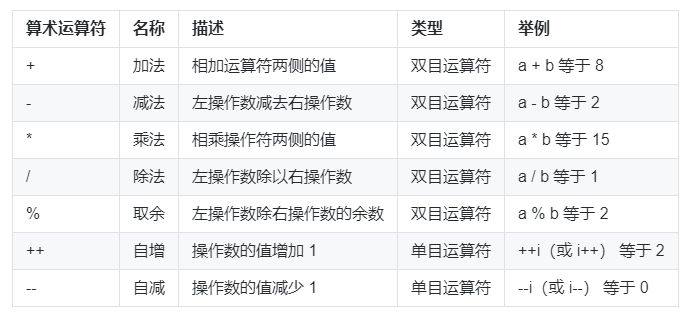其中，自增 (++) 和自减 (--) 运算符有两种写法：前缀（++i,--i）和后缀（i++,i--）

• 前缀自增自减法 (++i,--i): 先进行自增或者自减运算，再进行表达式运算。
• 后缀自增自减法 (i++,i--): 先进行表达式运算，再进行自增或者自减运算

新建一个源代码文件 ArithmeticOperation.java

public class ArithmeticOperation {
public static void main(String args[]) {
int a = 5;
int b = 3;
int c = 3;
int d = 3;
System.out.println("a + b = " + (a + b));
System.out.println("a - b = " + (a - b));
System.out.println("a * b = " + (a * b));
System.out.println("a / b = " + (a / b));
System.out.println("a % b = " + (a % b));
System.out.println("a++ = " + (a++));
System.out.println("++a = " + (++a));
System.out.println("b-- = " + (b--));
System.out.println("--b = " + (--b));
System.out.println("c++ = " + (c++));
System.out.println("++d = " + (++d));
}
}

编译运行：

$javac ArithmeticOperation.java$ java ArithmeticOperation
a + b = 8
a - b = 2
a * b = 15
a / b = 1
a % b = 2
a++ = 5
++a = 7
b-- = 3
--b = 1
c++ = 3
++d = 4

# 二、位运算符

Java 定义了位运算符，应用于整数类型 (int)，长整型 (long)，短整型 (short)，字符型 (char)，和字节型 (byte) 等类型。位运算时先转换为二进制，再按位运算。

表格中的例子中，变量 a 的值为 60（二进制：00111100），变量 b 的值为 13（二进制：00001101）：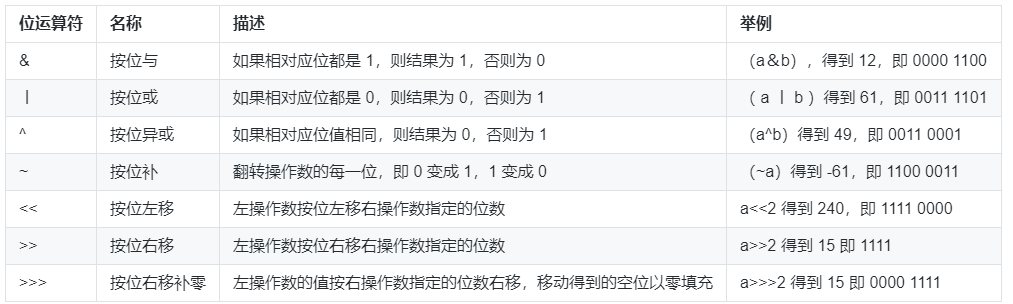新建一个源代码文件 BitOperation.java

public class BitOperation {
public static void main(String args[]) {
int a = 60;
int b = 13;
System.out.println("a & b = " + (a & b));
System.out.println("a | b = " + (a | b));
System.out.println("a ^ b = " + (a ^ b));
System.out.println("~a = " + (~a));
System.out.println("a << 2 = " + (a << 2));
System.out.println("a >> 2 = " + (a >> 2));
System.out.println("a >>> 2 = " + (a >>> 2));
}
}

编译运行：

$javac BitOperation.java$ java BitOperation
a & b = 12
a | b = 61
a ^ b = 49
~a = -61
a << 2 = 240
a >> 2 = 15
a >>> 2 = 15

# 三、逻辑运算符

逻辑运算符是通过运算符将操作数或等式进行逻辑判断的语句。

表格中的例子中，假设布尔变量 a 为真（true），变量 b 为假（false）：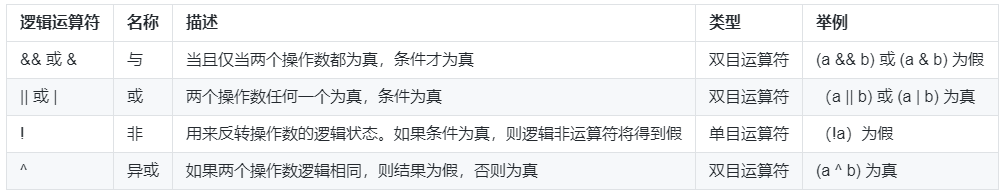&& 与 || 是具有短路性质，当按优先级顺序计算到当前表达式时，表达式的结果可以确定整个表达式的结果时，便不会继续向后进行判断和计算，而直接返回结果。

例如：当使用 && 逻辑运算符时，在两个操作数都为 true 时，结果才为 true，但是当得到第一个操作为 false 时，其结果就必定是 false，这时候就不会再判断第二个操作了。在计算表达式 (a & b) && (a | b) 时，首先计算 a & b 得到了 false，因为之后是 &&，任何值与 false 进行与操作都是 false，所以可以不用再计算下去，而直接返回 a & b 的结果 false

新建一个LogicOperation.java：

public class LogicOperation {
public static void main(String args[]) {
boolean a = true;
boolean b = false;
System.out.println("a && b = " + (a && b));
System.out.println("a || b = " + (a || b));
System.out.println("!a = " + (!a));
System.out.println("a ^ b = " + (a ^ b));
}
}

编译运行：

$javac LogicOperation.java$ java LogicOperation
a && b = false
a || b = true
!a = false
a ^ b = true

# 四、关系运算符

关系运算符生成的是一个 boolean（布尔）结果，它们计算的是操作数的值之间的关系。如果关系是真实的，结果为 true（真），否则，结果为 false（假）。

表格中的例子中，假设变量 a 3，变量 b 5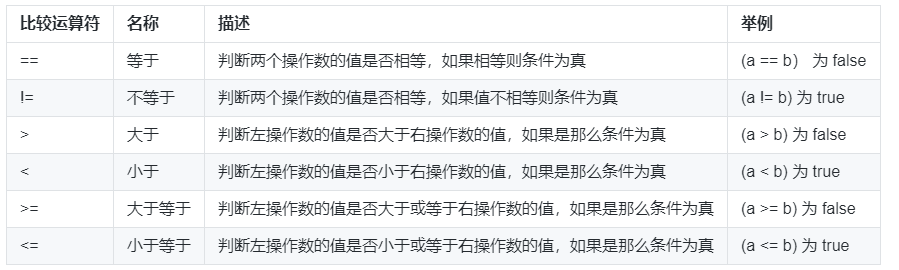除了上表列出的二元运算符，Java 还有唯一的一个三目运算符 ?:

语法格式：

布尔表达式 ？表达式 1 : 表达式 2;

运算过程：如果布尔表达式的值为 true，则返回表达式 1的值，否则返回表达式 2的值。

新建一个源代码文件 RelationalOperation.java

public class RelationalOperation {
public static void main(String args[]) {
int a = 3;
int b = 5;
System.out.println("a == b = " + (a == b));
System.out.println("a != b = " + (a != b));
System.out.println("a > b = " + (a > b));
System.out.println("a < b = " + (a < b));
System.out.println("a >= b = " + (a >= b));
System.out.println("a <= b = " + (a <= b));
System.out.println("a > b ? a : b = " + (a > b ? a : b));
}
}

编译运行：

$javac RelationalOperation.java$ java RelationalOperation
a == b = false
a != b = true
a > b = false
a < b = true
a >= b = false
a <= b = true
a > b ? a : b = 5

强调

• == 和 != 适用于所有的基本数据类型，其他关系运算符不适用于 boolean，因为 boolean 值只有 true 和 false，比较没有任何意义。
• == 和 != 也适用于所有对象，可以比较对象的引用是否相同。

引用：Java 中一切都是对象，但操作的标识符实际是对象的一个引用。

# 五、运算符优先级

运算符的优先级是帮助我们在一个表达式中如何对于不同的运算符和相同的运算符，进行正确的运算顺序。

运算符的优先级不需要特别地去记忆它，比较复杂的表达式一般使用圆括号 () 分开，提高可读性。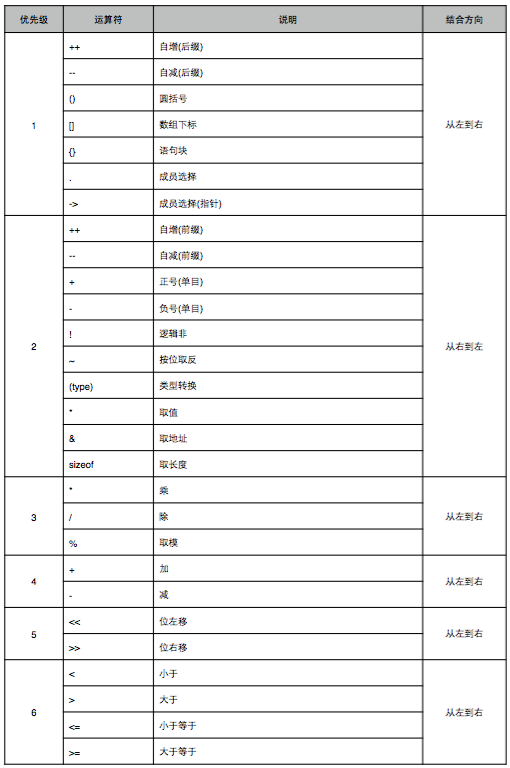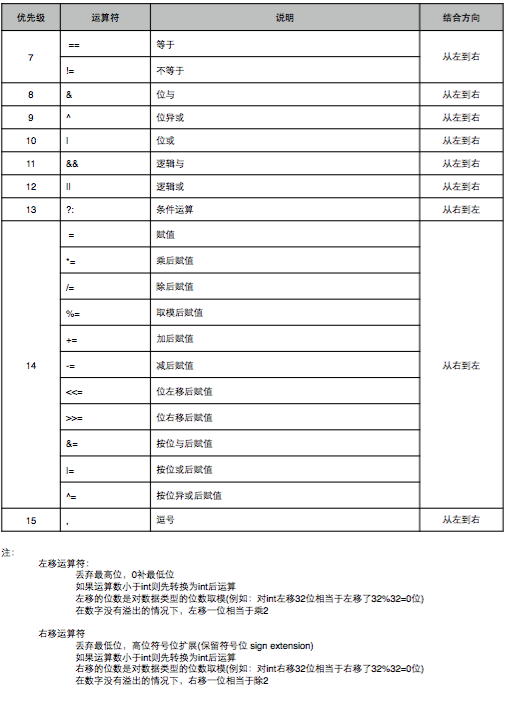参考资料：实验楼学习文档

展开全文java 操作符
• 文章目录算术运算符关系运算符逻辑运算符赋值运算符 算术运算符 算术运算符有：+ - * / % ++ – ++分为前置++ 和后置++，这俩者有所不同。 前置++是先加后用，后置++是先用后加。 前置–是先加后用，后置–是先用后...

# 算术运算符

算术运算符有：+ - * / % ++ –
++分为前置++ 和后置++，这俩者有所不同。
前置++是先加后用，后置++是先用后加。
前置–是先加后用，后置–是先用后加。
如下：我们可以看出++a在a++之前，也就是说 ++a和a++屏幕显示的是6。

public class s{
public static void main(String[] args)
{

int a,b;
a=5;b=15;
System.out.println(a+b);
System.out.println(a-b);
System.out.println(a*b);
System.out.println(a/b);
System.out.println(a%b);
System.out.println(++a);
System.out.println(a++);

}
}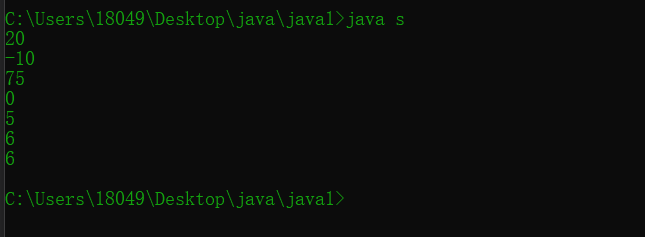## 关系运算符

关系运算符:> >= < <= == !=
关系运算符返回的值类型是boolean类型，即true和false

public class s{
public static void main(String[] args)
{

int a,b;
a=15;b=15;
System.out.println(a>b);
System.out.println(a>=b);
System.out.println(a<b);
System.out.println(a<=b);
System.out.println(a==b);
System.out.println(a!=b);

}
}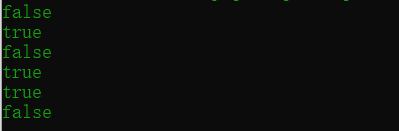### 逻辑运算符

逻辑运算符有： &（与） |（或） ^（异或） !（非）
&&(短路与) ||（短路或）
&&和&都是一假即假
||和|都是一真即真
^只有俩边不同才为真
！真变成假，假变成真
&&和&有所区别，当&前面的表达式为假的时候，后面的表达式依然会进行运算，而&&不会。

public class s{
public static void main(String[] args)
{

int a,b;
a=15;
b=15;
System.out.println(true&false);
System.out.println(true&true);
System.out.println(true|false);
System.out.println(true^false);
System.out.println(true^true);
System.out.println(!true);
System.out.println(a>b&&a++>b);
System.out.println(a);
System.out.println(a>b&a++>b);
System.out.println(a);

}
}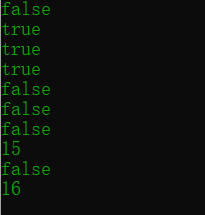可以看出&&后面的表达式并没有执行，所以a依然为15，
而&后面的表达式执行，a为16.

### 赋值运算符

赋值运算符：= += -= *= /= %=

public class s{
public static void main(String[] args)
{

byte a=10;
a=a+4;

}
}


这样的代码编译会报错，因为可能会损失精度，所以需要加强制类型转换。
而这样不会：

public class s{
public static void main(String[] args)
{

byte a=10;
a+=4;

}
}


这说明复合运算符会默认加上强制类型转换，即a=(byte)(a+4)。

展开全文javase
• 一、算术运算符 #define _CRT_SECURE_NO_WARNINGS #include <stdio.h> int main() { int a = 4 + 5 * 2 - 6 / 3 + 11 % 4;//4+10-2+3=15 printf("%d\n",a); scanf("%d", &a); printf("a=%d\n", a);...

目录

一、算术运算符

二、关系运算符

三、逻辑运算符

# 一、算术运算符

#define _CRT_SECURE_NO_WARNINGS
#include <stdio.h>

int main()
{
int a = 4 + 5 * 2 - 6 / 3 + 11 % 4;//4+10-2+3=15
printf("%d\n",a);
scanf("%d", &a);
printf("a=%d\n", a);//如果输入1234，输出4321
while (a != 0)
{
printf("%d", a % 10);
a = a / 10;
}
return 0;
}

# 二、关系运算符

#define _CRT_SECURE_NO_WARNINGS
#include <stdio.h>

int main()
{
int a = 2;
if (3 < a < 10)//无论a等于多少，都为真
{
printf("a is right");
}
else
{
printf("a is wrong");
}
return 0;
}

事实上，无论a等于多少，都会执行printf（“a is right”）;这条语句，因为两个<的优先级一致，所以从左到右按顺序执行

如果a大于3，结果为真（即1），1小于10，故总体为真；

如果a小于3，结果为假（即0），0小于10，故总体为真。

所以关系运算符不能这样用，如果要表示a>3同时a<10，要使用逻辑运算符

# 三、逻辑运算符

将上面的代码修改成

#define _CRT_SECURE_NO_WARNINGS
#include <stdio.h>

int main()
{
int a = 2;
if (3 < a && a < 10)
{
printf("a is right");
}
else
{
printf("a is wrong");
}
return 0;
}

练习：判断两个浮点数是否相等

写下面一段代码

#define _CRT_SECURE_NO_WARNINGS
#include <stdio.h>

int main()
{
float f = 234.56;

return 0;
}

进行调试，可以知道内存中f其实存的并不是准确的234.56，如图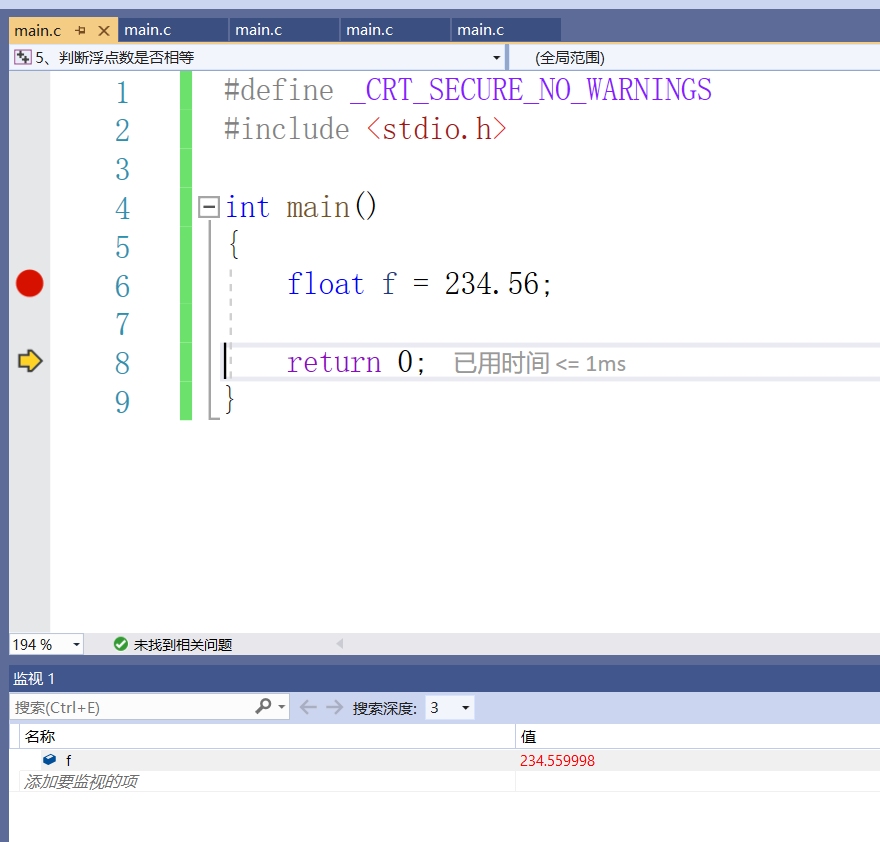所以如果写下面的代码不能进行正确的判断

#define _CRT_SECURE_NO_WARNINGS
#include <stdio.h>

int main()
{
float f = 234.56;
if (f == 234.56)
{
printf("f is equal to 234.56\n");
}
else
{
printf("f is not equal to 234.56");
}
return 0;
}

结果如下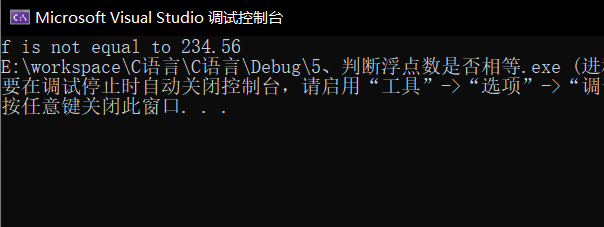所以必须用下面这种方法进行判断，原理是浮点数的精确度是7位

#define _CRT_SECURE_NO_WARNINGS
#include <stdio.h>

int main()
{
float f = 234.56;
if (f - 234.56 > -0.0001 && f - 234.56 < 0.0001)
{
printf("f is equal to 234.56\n");
}
else
{
printf("f is not equal to 234.56");
}
return 0;
}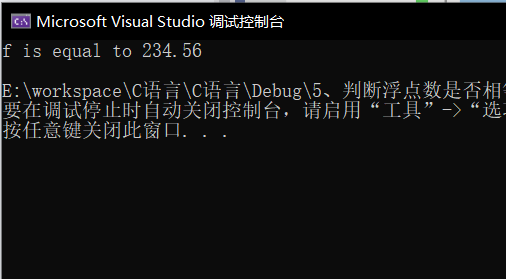练习：用c%输出0-128之间的整型数

错误代码

#define _CRT_SECURE_NO_WARNINGS
#include <stdio.h>

int main()
{
char a;
scanf("%d", &a);
printf("%c\n", a);
return 0;
}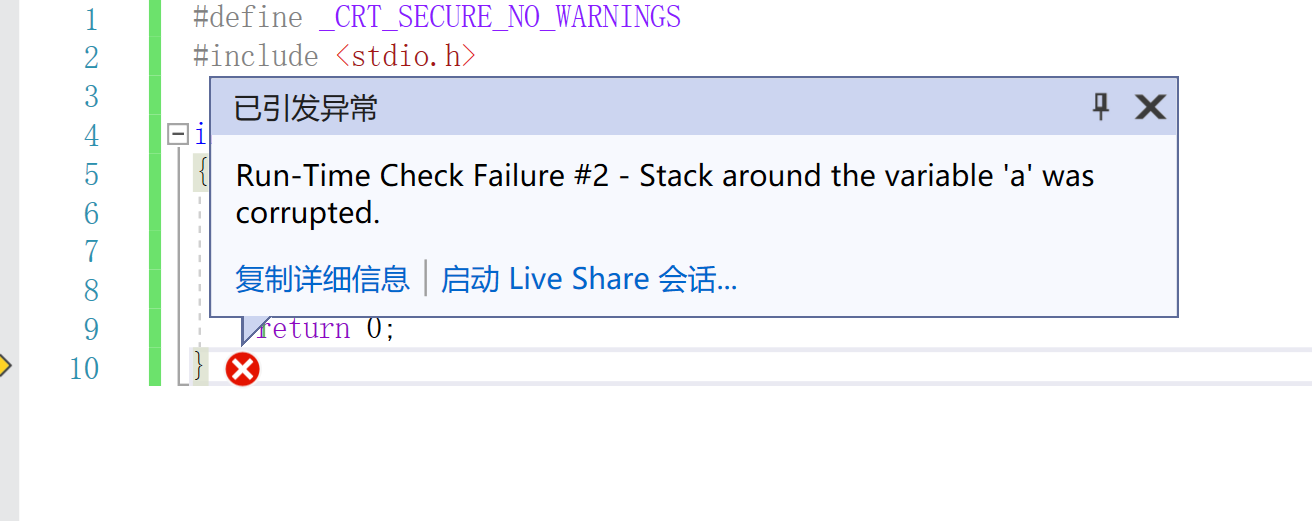原因：由于char占1个字节，int占四个字节，输入四个字节的数，要求输出char类型的a（1个字节）超出空间。

正确代码

#define _CRT_SECURE_NO_WARNINGS
#include <stdio.h>

int main()
{
int a;
scanf("%d", &a);
printf("%c\n", a);
return 0;
}

此时的a也是四个字节的整型，0-128之间的整型数可以用c%输出。

展开全文c语言 开发语言 后端
• Java的关系运算符(relational operators)也称为“比较运算符”，用于用来比较判断两个变量或常量的大小。关系运算符是二元运算符，运算结果是 boolean 型。当运算符对应的关系成立时，运算结果是 true，否则是 false...
• 关系运算符： 如图所示，关系运算符是比较大小的运算符。分优先级高和低，优先级高的要比优先级低的先运算。 关系运算符获得的是一个逻辑值，只有真或者假，C语言中的逻辑值用1来表示真，用0来表示假。 但C语言...c语言
• ## Java关系运算符

千次阅读 2021-02-26 09:56:33
Java关系运算符java里的关系运算符有这么几种：大于(>)、小于(=)、小于等于(<=)，他们运算的结果是个boolean值，关系式成立为true，不成立为false。运算符分类编号关系运算符说明1>大于2<小于3==等于4!=...
• 上期知识回顾 自增自减运算符(练习) //自增自减运算符 y的值是多少? int x = 10; int y = x++ ＋ x++ + x++;...注意事项:关系运算符的结果都是boolean类型，要么是true 要么是false。千万不要把’'误写成’=java
• 算术运算符、关系运算符、逻辑运算符、赋值运算符详解与优先级关系一、算术运算符二、关系运算符三、逻辑运算符四、赋值运算符 四种运算符优先级关系： 算术运算符>关系运算符>逻辑运算符>赋值运算符 一...
• 1.关系运算符用来比较运算。关系运算的结果是布尔值：true/false； == 等于 ！= 不等于 < 小于 > 大于 >= 大于或等于 <= 小于或等于 2.注意事项 = 是赋值运算符，而真正判断两个操作数是否相等...java
• 赋值运算符=，把等号右边的数据赋值给等号左边的变量或常量+=，a += b，等效于：a = (a的数据类型)(a + (b));-=，a -= b，等效于：a = (a的数据类型)(a - (b));=，a= b，等效于：a = (a的数据类型)(a * (b));/=，a /...
• 关系运算符在使用时，它的的两边都会有一个表达式，比如变量、数值、加减乘除运算等，关系运算符的作用就是判明这两个表达式的大小关系。注意，是判明大小关系，不是其他关系。C语言提供了以下关系运算...
• 关系运算符用于判断两个变量或常量的大小，运算结果是一个布尔类型的值true或false。 > ：大于 >= ：大于等于 < ：小于 <= ：小于等于 == ：等于 != ：不等于 int a = 1; int b = 1; ...java 开发语言
•linux shell 运维
• package 运算符; public class Demo3 {//运算符 ... * 关系运算符:>,<,>=,<=,==,!=(不等于) * 逻辑运算符:&&(与),||(或),!(非) 与或非 * 位运算符:&,|,^,~,>>,<<,>>>.java
• c语言中的关系运算符和逻辑运算符1. 逻辑运算符逻辑运算符是指用形式逻辑原则来建立数值间关系的符号。Turbo C的逻辑运算符如下:━━━━━━━━━━━━━━━━━━━━━━━━━━━━━操作符 作用─────...
• 本来今天是准备写if函数的，结果发现关系运算符没教你们可能会看不懂，所以今天就先讲关系运算符 今天主要讲解的关系运算符有： == , < , >, <= , >= , != 关系运算符得到的是一个布尔值（真或假），...c++ 开发语言 后端
• ### 【附录2：算术运算符、关系运算符、逻辑运算符】 文章里面的所有截图和“之前所学过的内容”都是来自于翁恺的C语言视频。 之前已经学习过简单的算术运算符、关系运算符（又称比较运算符）、逻辑运算符。为了...c语言 经验分享
• 算术和关系运算符 一、常见的运算符 1、算术运算符： + - * /(除）（c语言中叫整除，并不是真正的除法） %（取余）（余数没0，说明能整除） //（整除）地板除法 “**”（幂...python
• 4.1关系运算符和逻辑运算符 您好，前面我们介绍了顺序结构程序，顺序结构程序的特点，就是按照从上到下的顺序依次的来执行程序中的语句，而实际中我们编写的程序，往往要对很多复杂的情况进行处理，这样就需要用到...
• 赋值运算符、算术运算符、关系运算符、逻辑运算符、条件运算符（三元运算符）、位运算符、运算符的优先级java
• ## 比较、关系运算符

千次阅读 2020-11-30 22:34:00
比较(即关系，条件)运算符python中的比较运算符如下表| 运算符 | 描述 | 示例 || --- | --- | --- || \== | 检查两个操作数的值是否相等，如果是则条件变为真。 | 如a=3,b=3，则(a == b) 为 True || != | 检查两个...
• 关系运算符主要是完成数据和数据之间的比较，比如：5>3，结果是 true（真），5>10，结果是 false（假），那么关系运算符都有哪些呢？>、>=、<、<=、==、!=。关系运算符是比较简单容易理解的，我们...
• 赋值、关系运算符 一、赋值运算符 (一)、基本的赋值运算符是=,一开始可能会以为它是“等于”，其实不是的,可以读作"等于" (二)、赋值运算符实际上意味着把等号右边的值或表达式的值赋给等号左边 (三)、运算符 1、+=...javascript...